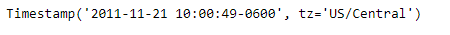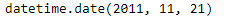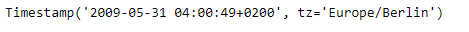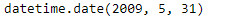GeeksforGeeks App
Open AppBrowser
Continue

# Python | Pandas Timestamp.date

Python is a great language for doing data analysis, primarily because of the fantastic ecosystem of data-centric python packages. Pandas is one of those packages and makes importing and analyzing data much easier.

Pandas` Timestamp.date()` function return a datetime object with same year, month and day as that of the given Timestamp object.

Syntax : Timestamp.date()

Parameters : None

Return : date

Example #1: Use `Timestamp.date()` function to return the date of the given Timestamp object as a datetime object.

 `# importing pandas as pd``import` `pandas as pd`` ` `# Create the Timestamp object``ts ``=` `pd.Timestamp(year ``=` `2011``,  month ``=` `11``, day ``=` `21``,``           ``hour ``=` `10``, second ``=` `49``, tz ``=` `'US/Central'``)`` ` `# Print the Timestamp object``print``(ts)`

Output :Now we will use the `Timestamp.date()` function to return the date as a datetime object.

 `# return as a datetime object ``ts.date()`

Output :As we can see in the output, the `Timestamp.date()` function has returned a datetime object containing the same year, month and day as that of the given Timestamp object.

Example #2: Use `Timestamp.date()` function to return the date of the given Timestamp object as a datetime object.

 `# importing pandas as pd``import` `pandas as pd`` ` `# Create the Timestamp object``ts ``=` `pd.Timestamp(year ``=` `2009``,  month ``=` `5``, day ``=` `31``,``        ``hour ``=` `4``, second ``=` `49``, tz ``=` `'Europe/Berlin'``)`` ` `# Print the Timestamp object``print``(ts)`

Output :Now we will use the `Timestamp.date()` function to return the date as a datetime object.

 `# return as a datetime object ``ts.date()`

Output :As we can see in the output, the `Timestamp.date()` function has returned a datetime object containing the same year, month and day as that of the given Timestamp object.

My Personal Notes arrow_drop_up